Question
Part A and Part B.
Also question #3-post lab. (its circled)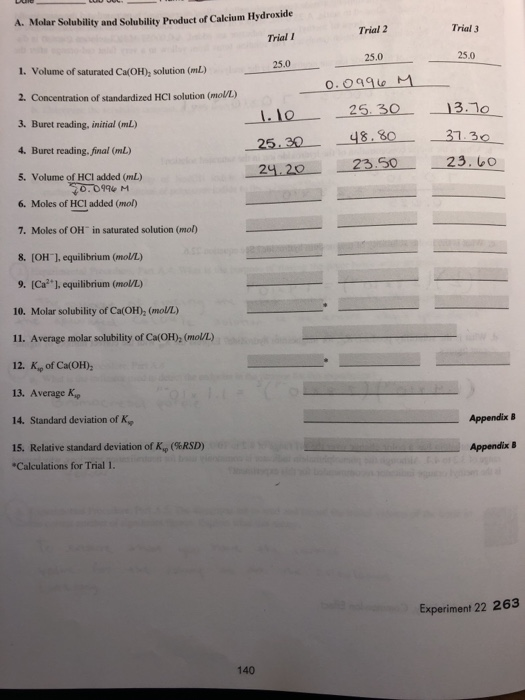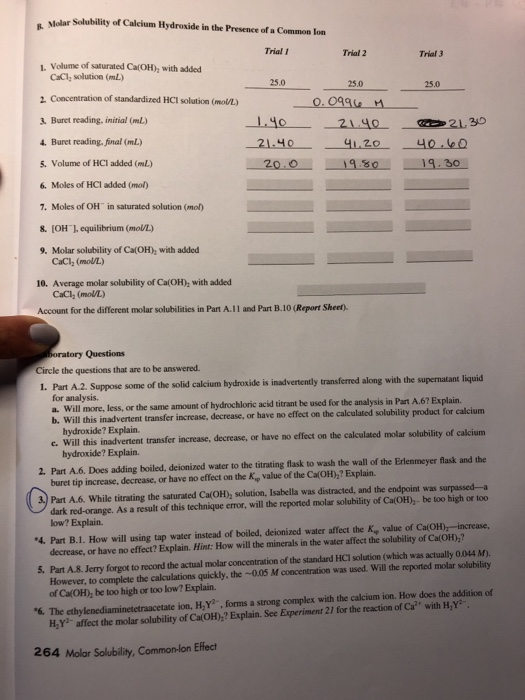A. Molar Solubility and Solubility Product of Calcium Hydroxide Trial I Trial 3 Trial 2 25.0 1. Volume of saturated Ca(OH), solution (mL) 2. Concentration of standardized HCl solution (molU/L) 3. Buret reading, initial (mL 4. Buret reading, final (mL) 5. Volume of HCI added (mL) 6. Moles of HCI added (mol) 7. Moles of OH" in saturated solution (mol) 8. (OH1, equilibrium (mol/L) 9. (Ca2 ], equilibrium (mol/L) 25.0 11 25313.1 25.30 48.3031.3 10. Molar solubility of Ca(OH)2 (moU/L) 11. Average molar solubility of Ca(OH), (mol/L) 12. Kio of Ca(OH) 13. Average Kp 14. Standard deviation of K, 15. Relative standard deviation of K, (%RSD) Appendix B Appendix B Calculations for Trial 1. Experiment 22 263 140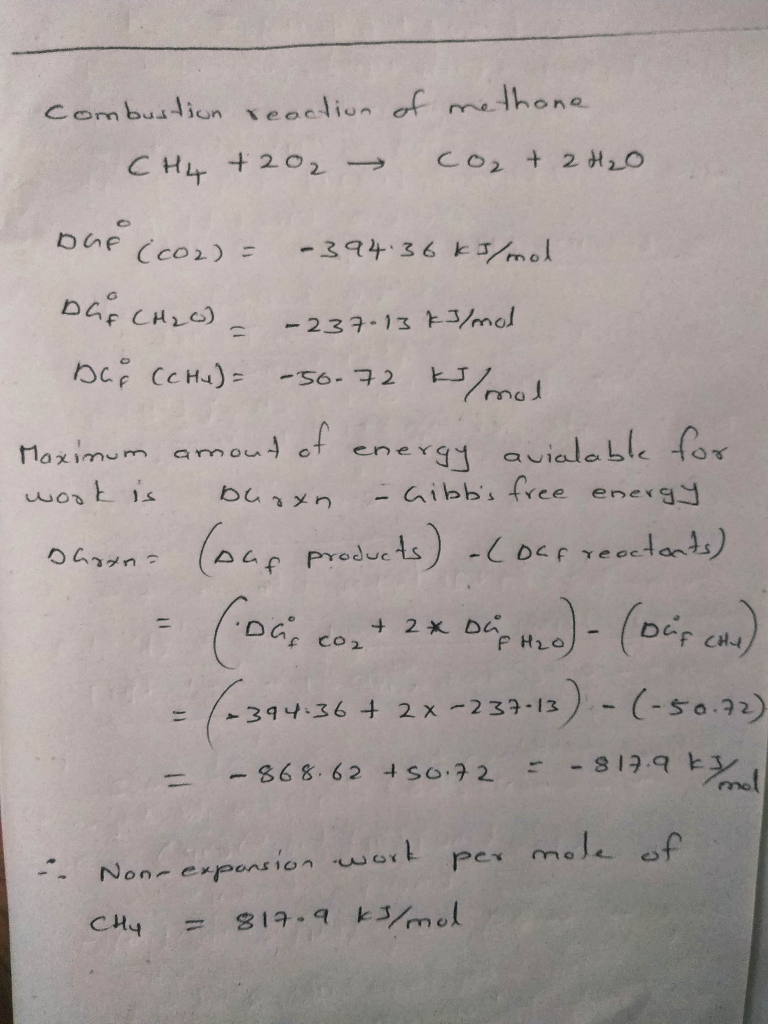#### Earn Coins

Coins can be redeemed for fabulous gifts.

Similar Homework Help Questions
• ### Isnt the molar solubility of Ca(OH)2 with added CaCl2 supposed to be less than the molar solubili...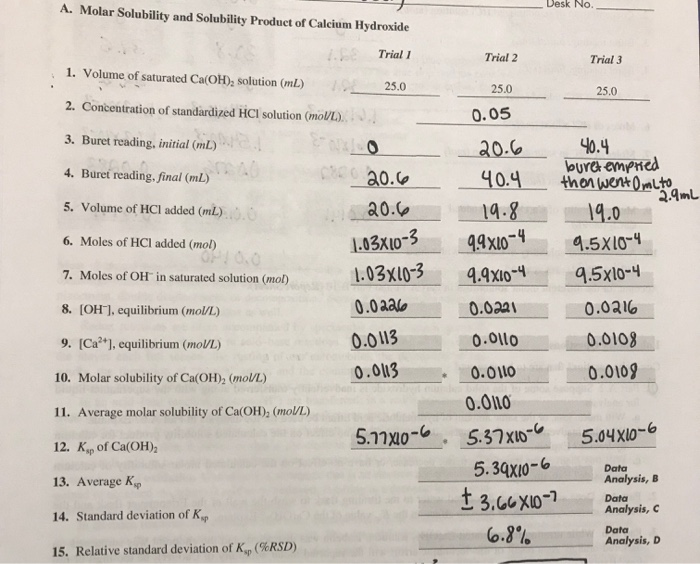isnt the molar solubility of Ca(OH)2 with added CaCl2 supposed to be less than the molar solubility of Ca(OH)2 by itself? where did I mess up? A. Molar Solubility and Solubility Product of Calcium Hydroxide メーーー Desk No. Trial 1 Trial 2 Trial 3 1. Volume of saturated CalOHD, solution (ml) 2. Concentration of standardized HCI solution (mol/L) 3. Buret reading, initial (mL) . 4. Buret reading,final(mL) 5. Volume of HCI added (mL) 6. Moles of HCl added (mol) 7....

• ### Molar Solubility and Solubility Product of Calcium Hydroxide Volume of saturated Ca(OH)2 solution (mL) 25.00...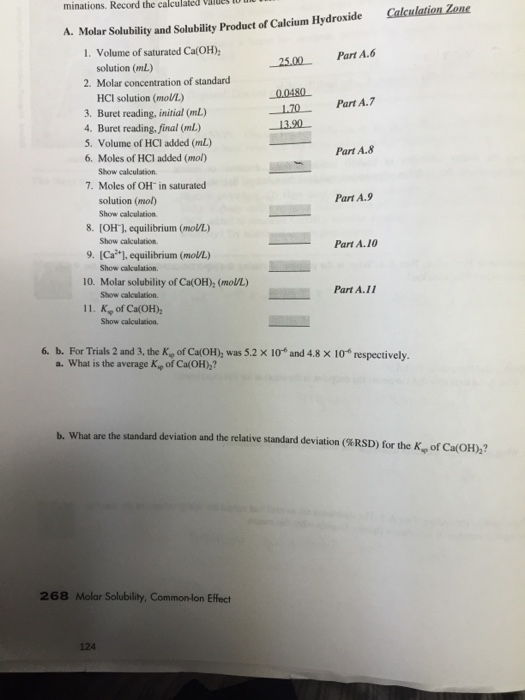Molar Solubility and Solubility Product of Calcium Hydroxide Volume of saturated Ca(OH)2 solution (mL) 25.00 Molar concentration of standard HCI solution (mol/L.) 0.0480 Buret reading, initial (mL) 1.70 Buret reading, final (mL) 13.90 Volume of HCI added (mL) Moles of HCI added (mol) Show calculation Moles of OH^- in saturated solution (mol) Show calculation [OH^-), equilibrium (mol/L) Show calculation. [Ca^2+], equilibrium (mol/L) Show calculation. Molar solubility of Ca(OH)2 (mol/L) Show calculation. Ks rho of Ca(OH)2 Show calculation. For Trials 2...

• ### A determination the molar solubility and the K_sp calcium hydroxide was completed according to the experimenta...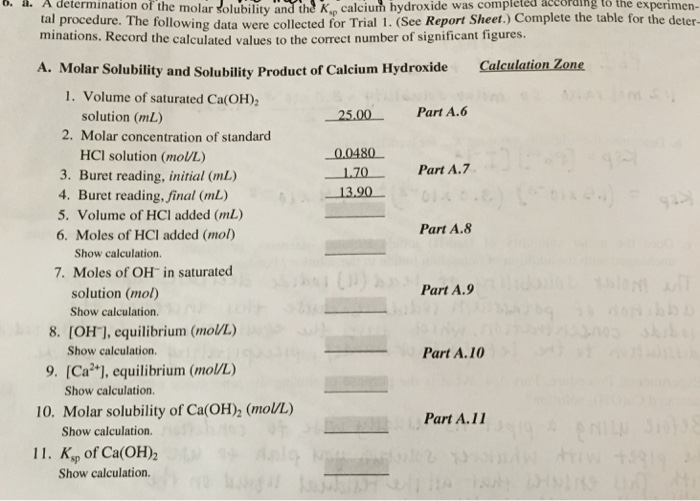A determination the molar solubility and the K_sp calcium hydroxide was completed according to the experimental procedure. The following data were collccicd for Trial 1 (Sec Repon sheet. minations. Record the calculated values to the correct number of significant ligures. A. Molar Solubility and Solubility Product of Calcium Hydroxide 1.Volume of saturated Ca(OH)_2 solution (mL) 2.Molar concentration of standard HCI solution (mol/L) 3.Buret reading, initial (mL) 4.Buret reading, final (mL) 5.Volume of HCI added (mL) 6.Moles of HCI added (mol)...

• ### Trial 1 Data 1. Volume of saturated Ca(OH)2 solution (mL) 25.00 2. Molar concentration of standard...

Trial 1 Data 1. Volume of saturated Ca(OH)2 solution (mL) 25.00 2. Molar concentration of standard HCl solution (mL) 0.0480 3. Buret reading, initial (mL) 1.70 4. Buret reading, final (mL) 13.90 Calculate the molar solubility and Ksp for calcium hydroxide based on the table information provided. Molar Solubility Ksp For trials 2 and 3, the Ksp of Ca(OH)2 was and respectively. What is the percent relative standard deviation (%RSD) of the three trials?

• ### 1. The Molar Solubility of Ca(10), in Pure Water Temperature of the saturated solution of calcium...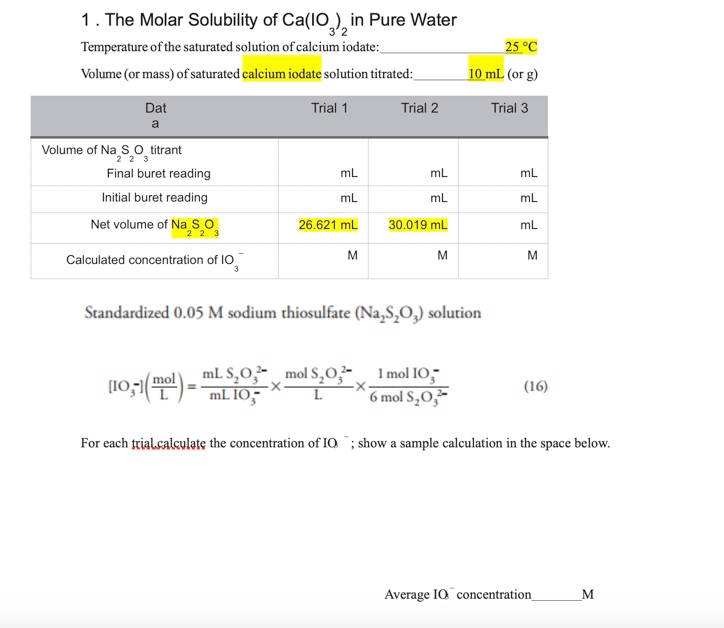1. The Molar Solubility of Ca(10), in Pure Water Temperature of the saturated solution of calcium iodate: Volume (or mass) of saturated calcium iodate solution titrated: 25 °C 10 ml (or g) Dat Trial 1 Trial 2 Trial 3 Volume of Na S o titrant Final buret reading Initial buret reading ml mL mL mL Net volume of Na So 26.621 mL 30.019 mL Calculated concentration of 10 Standardized 0.05 M sodium thiosulfate (Na,8,0,) solution (10,51(E) - TIL 10 mols.0.2...

• ### Please help me with 6a calculations Experimental Procedure, Part A.6 versus Part B.2. Would you expect more or...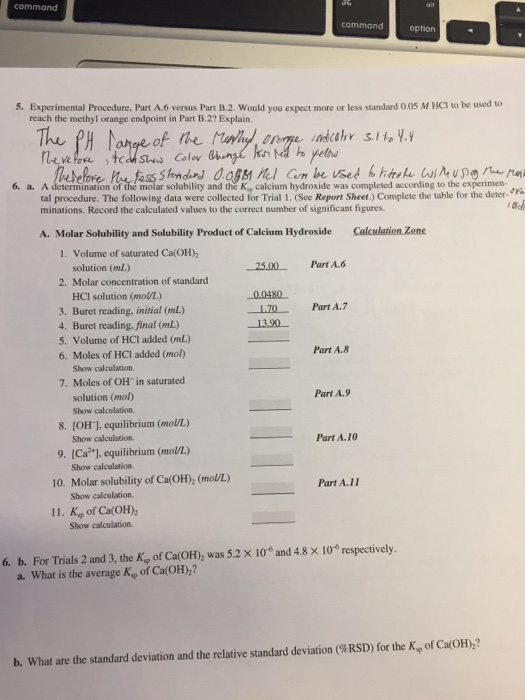Please help me with 6a calculations Experimental Procedure, Part A.6 versus Part B.2. Would you expect more or less standard 0.05 M HCI to be used to reach the methyl orange endpoint in Part B.2? Explain. A determination of the molar solubility and the K_sp calcium hydroxide was completed according to the experimental procedure. The following data were collected for Trial 1. (See Report Sheet.) Complete the table for the determinations. Record the calculated values to the correct number of...

• ### 1. The Molar Solubility of Ca(10), in Pure Water Temperature of the saturated solution of calcium...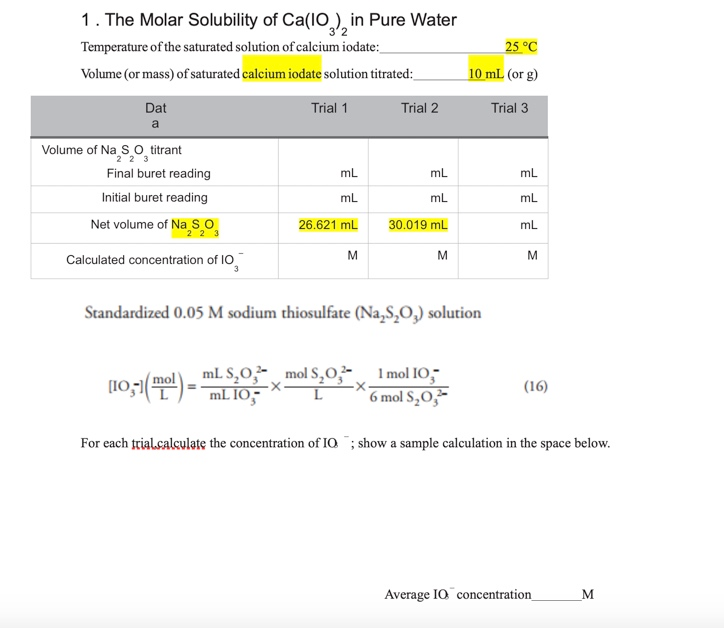1. The Molar Solubility of Ca(10), in Pure Water Temperature of the saturated solution of calcium iodate: Volume (or mass) of saturated calcium iodate solution titrated: 25 °C 10 ml (or g) Dat Trial 1 Trial 2 Trial 3 Volume of Na S o titrant Final buret reading Initial buret reading ml mL mL mL Net volume of Na So 26.621 mL 30.019 mL Calculated concentration of 10 Standardized 0.05 M sodium thiosulfate (Na,8,0,) solution (10,51(E) - TIL 10 mols.0.2...

• ### Partner Data Molarity of HCI solution: A. Saturated Ca(OH) at Room Temperature Temperature of calcium hydroside...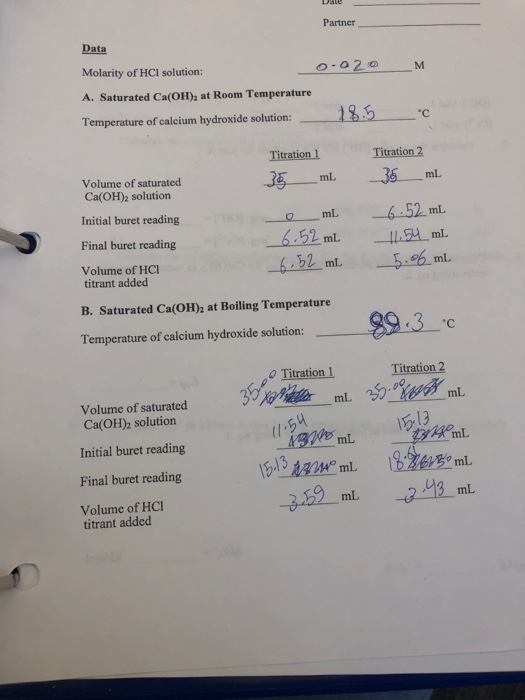Partner Data Molarity of HCI solution: A. Saturated Ca(OH) at Room Temperature Temperature of calcium hydroside solution:1.5 c Titration 1 Volume of saturated Ca(OH)2 solution Initial buret reading Final buret reading Volume of HCI mL. 2 ml titrant added B. Saturated Ca(OH)2 at Boiling Temperature Temperature of calcium hydroxide solution: --S9.3. Titration 1 Volume of saturated Ca(OH)2 solution 13 il Initial buret reading 例3mno mL8segomi. Final buret reading mL Volume of HCI titrant added We were unable to transcribe this...

• ### Suppose some of the solid calcium hydroxide is inadvertently transferred along with the supernatant liquid for...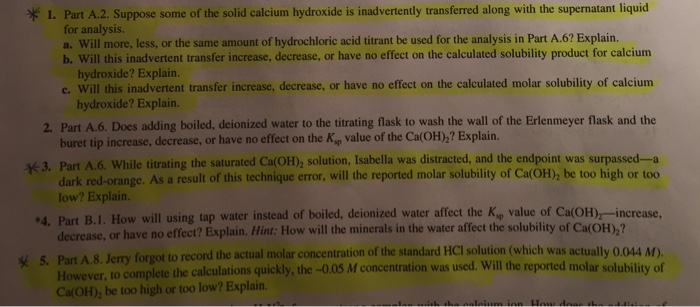Suppose some of the solid calcium hydroxide is inadvertently transferred along with the supernatant liquid for analysis. a.Will more. less, or the same amount of hydrochloric acid titrant be used for the analysis in .6? Explain. b.Will this inadvertent transfer increase, decrease, or have no effect on the calculated solubility product for calcium hydroxide? Explain. c.Will this inadvertent transfer increase, decrease, or have no effect on the calculated molar solubility of calcium hydroxide? Explain. 2. .6 Does adding beiled, deionized...

• ### Solubility product constant of calcium iodate/common ion effect. I am unsure how to do this however...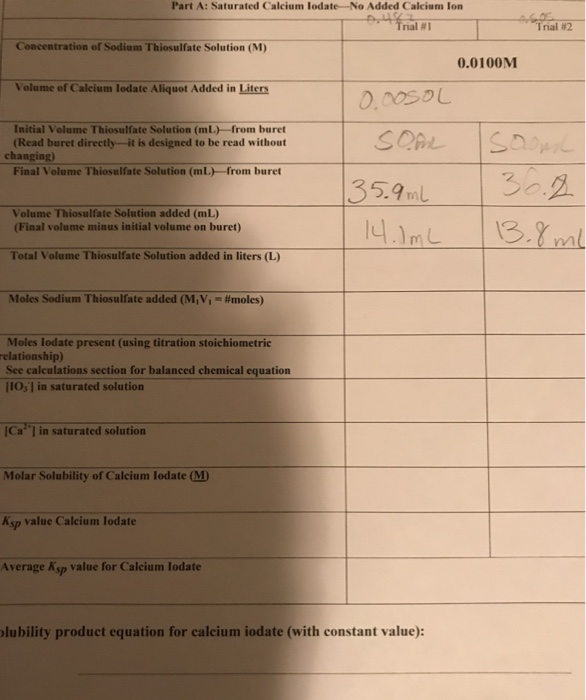Solubility product constant of calcium iodate/common ion effect. I am unsure how to do this however I am only asking for help on trial one as there are 4 total trials and I would like to be able to do those on my own after I have learned how to do the work. Thanks. Also, could you provide the balanced equation for the titration as well as the solubility equation for iodate? Part A: Saturated Calcium lodate-No Added Calcium lon...